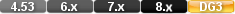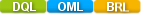Easy to Create, Easy to Change - Easy to use!## InstallmentInstallment(PRESENTVALUE,FUTUREVALUE,RATE,PERIODE) Installment(NUMERIC VALUE,NUMERIC VALUE,NUMERIC VALUE,NUMERIC VALUE) Installment(15000,0,10/12,60)

The installment function calculates the periodic installment payment required for a financial transaction given the presentvalue, futurevalue, interest rate, and number of payment periods.

If the installment payment increases the value of the investment (e.g., an annuity), the numeric value returned by the function is expressed as a positive amount. If the payment decreases the value of the investment (e.g., loan amortization), the numeric value returned by the function is expressed as a negative amount. The interest rate, installment payments, and time periods must be based on the same terms. For example, if payments are made monthly on a five-year loan, the number of periods is (12 * 5) or 60. The interest rate must be expressed in the same terms (the monthly interest rate is the annual rate /12). When typing fractions like 10 / 12, DataEase requires that you type a space before and after the / division symbol.

# Parameters

PRESENTVALUE

The value of the financial instrument today.

FUTUREVALUE

The value of the financial instrument at the end of the period.

RATE

The interest rate paid in the periode

PERIODE

Periode

# Returns/Result

A numeric value (the installment payment amount).

# Examples

Example 1

installment( 15000, 0, 10 / 12, 60 )

Returns:-318.71

This example calculates the monthly installment payment required to pay off a \$15,000 loan in 5 years at an annual interest rate of 10%.

Example 2

installment( 0, 15000, 10 / 12, 60)

Returns:193.71

This example calculates the monthly installment amount required to accumulate \$15,000 in 5 years at an annual interest rate of 10%.

Note: DataEase financial functions are derived from the formula shown below. (The double asterisks (meaning "raise to the power") cannot be used in a script).

futurevalue = principal * ((1 + (rate/100))) ** periods) + (installment/(rate/100)) * (((1 + (rate/100)) ** periods) - 1)

# installment

#### Type

Financial Function

#### Purpose

The installment function calculates the periodic installment payment required for a financial transaction given the presentvalue, futurevalue, interest rate, and number of payment periods.

Syntax

installment( presentvalue, futurevalue, rate, periods)

Returns

A numeric value (the installment payment amount).

Usage

If the installment payment increases the value of the investment (e.g., an annuity), the numeric value returned by the function is expressed as a positive amount. If the payment decreases the value of the investment (e.g., loan amortization), the numeric value returned by the function is expressed as a negative amount. The interest rate, installment payments, and time periods must be based on the same terms. For example, if payments are made monthly on a five-year loan, the number of periods is (12 * 5) or 60. The interest rate must be expressed in the same terms (the monthly interest rate is the annual rate /12). When typing fractions like 10 / 12, DataEase requires that you type a space before and after the / division symbol.

#### Example 1

installment( 15000, 0, 10 / 12, 60 )

Returns:-318.71

This example calculates the monthly installment payment required to pay off a \$15,000 loan in 5 years at an annual interest rate of 10%.

Example 2

installment( 0, 15000, 10 / 12, 60)

Returns:193.71

This example calculates the monthly installment amount required to accumulate \$15,000 in 5 years at an annual interest rate of 10%.

Note: DataEase financial functions are derived from the formula shown below. (The double asterisks (meaning "raise to the power") cannot be used in a script).

futurevalue = principal * ((1 + (rate/100))) ** periods) + (installment/(rate/100)) * (((1 + (rate/100)) ** periods) - 1)